# RC filters, integrators and differentiatorsFrom Physclips: Mechanics with animations and film.

RC circuits work as filters (high-pass or low-pass filters), integrators and differentiators. Here we explain how, and give sound files examples of RC filters in action. For an introduction to AC circuits, resistors and capacitors, see AC circuits.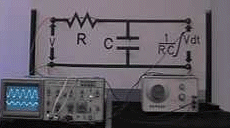A triangle wave (upper trace) is integrated to give a rounded, parabolic wave.

Overview. A low pass filter passes low frequencies and rejects high frequencies from the input signal. And vice versa for a high pass filter. The simplest of these filters may be constructed from just two low-cost electrical components. Over appropriate frequency ranges, these circuits also integrate and differentiate (respectively) the input signal.

### Low pass filter

A first order, low pass RC filter is simply an RC series circuit across the input, with the output taken across the capacitor. We assume that the output of the circuit is not connected, or connected only to high impedance, so that the current is the same in both R and C.The voltage across the capacitor is IXC = I/ωC. The voltage across the series combination is IZRC = I(R2 + (1/ωC)2)1/2, so the gain is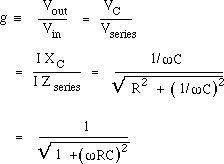From the phasor diagram for this filter, we see that the output lags the input in phase.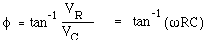At the angular frequency ω = ωo = 1/RC, the capacitive reactance 1/ωC equals the resistance R. We show this characteristic frequency* on all graphs on this page. For instance, if R = 1 kω and C = 0.47 mF, then 1/RC = ωo = 2.1 103 rad.s-1, so fo = ωo/2π = 340 Hz.

* Let's check the units: it should be quick. First, a radian is the ratio of an arc to a radius, so it is a pure number. R is in ohms, and we can use Ohm's law (V = IR) and the definition of current (I = dQ/dt) to write ohm = volt/ampere = volt/(coulomb/second) = volt*second/coulomb. C (charge per unit voltage) is in farads (coulomb/volt), so RC has the units of (volt*second/coulomb)(coulomb/volt) = second. See, it was quick: it only took us 30 ohm.farads!

At this frequency, the gain = 1/20.5 = 0.71, as shown on the plot of g(ω). The power transmitted usually goes as the gain squared, so the filter transmits 50% of maximum power at fo. Now a reduction in power of a factor of two means a reduction by 3 dB (see What is a decibel?). A signal with frequency f = fo = 1/2πRC is attenuated by 3 dB, lower frequencies are less attenuated and high frequencies more attenuated.

At ω = ωo, the phase difference is π/4 radians or 45°, as shown in the plot of φ(ω).

### High pass filter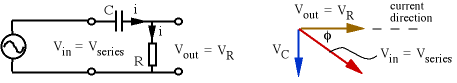The voltage across the resistor is IR. The voltage across the series combination is IZRC = I(R2 + (1/ωC)2)1/2, so the gain is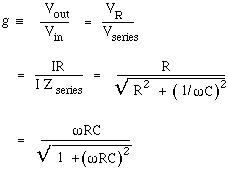From the phasor diagram for this filter, we see that the output leads the input in phase.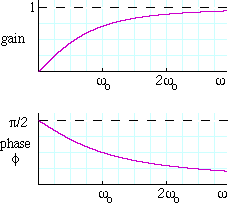Once again, when w = ωo = 1/RC, the gain = 1/20.5 = 0.71, as shown on the plot of g(ω). A signal with frequency f = fo = 1/2πRC is attenuated by 3 dB, higher frequencies are less attenuated and lower frequencies more attenuated. Again, when ω = ωo, the phase difference is π/4 radians or 45f.

### Filter applications and demonstrations

RC and other filters are very widely used in selecting signals (which are voltage components one wants) and rejecting noise (those one doesn't want). A low pass filter can 'smooth' a DC power supply: allow the DC but attenuate the AC components. Conversely, a high pass filter can pass the signal into and out of a transistor amplifier stage without passing or affecting the DC bias of the transistor. They can also be used to sort high frequency from low frequency components in a purely AC signal. Capacitors are often used in 'cross over' networks for loudspeakers, to apply the high frequencies to the 'tweeter' (a small, light speaker) and the low frequencies to the 'woofer' (a large, massive speaker). We include some sound examples here as demonstrations.

 No filter. First we recorded the sound from a microphone into the computer sound card, without any (extra*) filtering. * The sound coming out of your computer speakers will nevertheless have been filtered by the time you hear it. A speaker already filters the sound, because its impedance is partly inductive, due to the speaker coil. Further, its acoustic efficiency is a strong function of frequency. Nevertheless, you should notice the differences among these sound files, particularly if you switch from one to another in succession.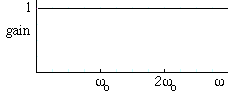Low pass filter. At high frequencies, the capacitor 'shorts out' the input to the sound card, but hardly affects low frequencies. So this sound is less 'bright' than the example above. This sound is quieter than the previous sample. We have cut out the frequencies above 1 kHz, including those to which your ear is most sensitive. (For more about the frequency response of the ear, see Hearing Curves.)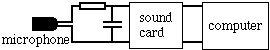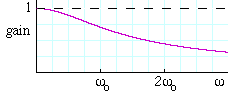High pass filter. At low frequencies, the reactance of the capacitor is high, so little current goes to the speaker. This sound is less 'bassy' than those above. Losing the low frequencies makes the sound rather thin, but it doesn't reduce the loudness as much as removing the high frequencies. (If you do not notice much difference with the high pass filter, it may be because you are using tiny computer speakers that do not radiate low frequencies well. Try it with headphones or with hifi speakers.)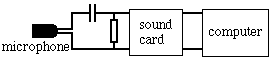Filger gains are usually written in decibels. see the decibel scale.

### Integrator

 Here we have an AC source with voltage vin(t), input to an RC series circuit. The output is the voltage across the capacitor. We consider only high frequencies ω >> 1/RC, so that the capacitor has insufficient time to charge up, its voltage is small, so the input voltage approximately equals the voltage across the resistor.The photograph at the top of this page shows a triangle wave input to an RC integrator, and the resulting output.

### Differentiator

 Again we have an AC source with voltage vin(t), input to an RC series circuit. This time the output is the voltage across the resistor. This time, we consider only low frequencies ω << 1/RC, so that the capacitor has time to charge up until its voltage almost equals that of the source.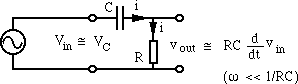More accurate integration and differentiation is possible using resistors and capacitors on the input and feedback loops of operational amplifiers. Such amplifiers can also be used to add, to subtract and to multiply voltages. An analogue computer is a combination of such circuits, and may be used to solve simultaneous, differential and integral equations very rapidly.

Go back to AC circuits

 Joe Wolfe / J.Wolfe@unsw.edu.au, phone 61- 2-9385 4954 (UT + 10, +11 Oct-Mar). School of Physics, University of New South Wales, Sydney, Australia. There are pages on related material at### Happy birthday, theory of relativity!

As of June 2005, relativity is 100 years old. Our contribution is Einstein Light: relativity in brief... or in detail. It explains the key ideas in a short multimedia presentation, which is supported by links to broader and deeper explanations.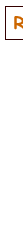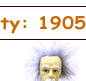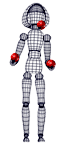This work is licensed under a Creative Commons License.